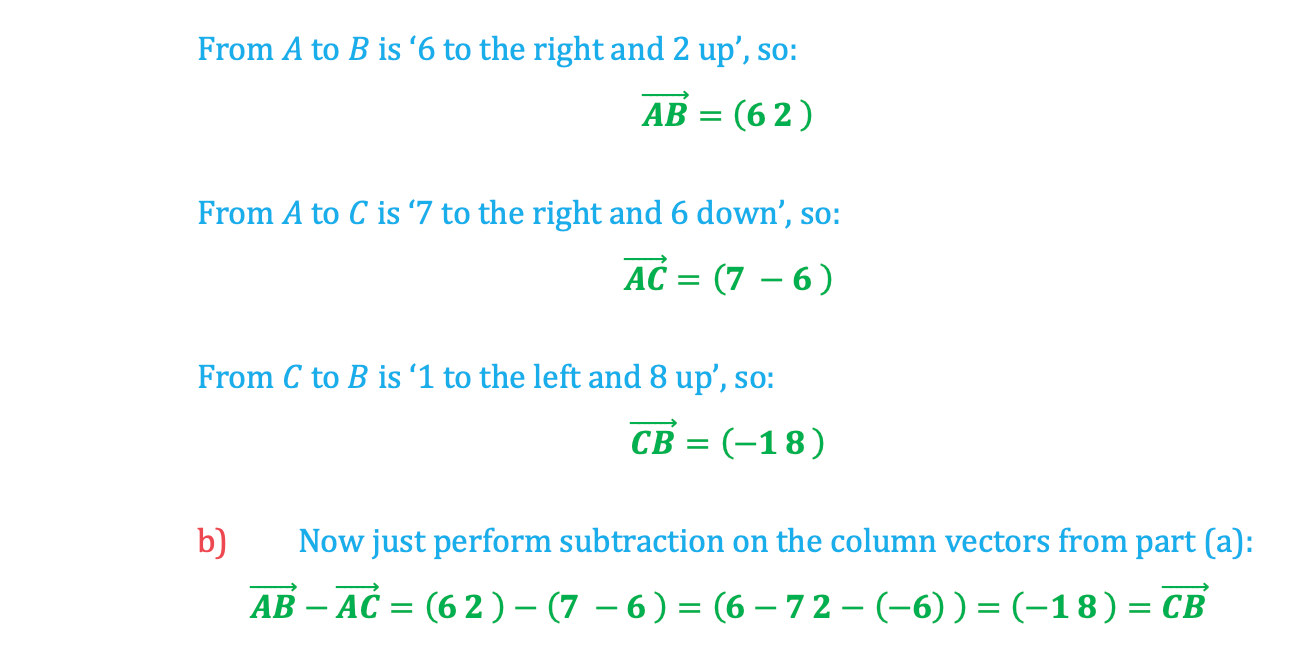# Edexcel IGCSE Maths 复习笔记 5.1.1 Vectors - Basics

Edexcel IGCSE Maths 复习笔记 5.1.1 Vectors - Basics

#### What are vectors?

• vector is a type of number that has both a size and a direction
• At GCSE we only deal with two-dimensional vectors, although it is possible to have vectors with any number of dimensions

#### Representing vectors

• Vectors are represented as arrows, with the arrowhead indicating the direction of the vector, and the length of the arrow indicating the vector’s magnitude (ie its size):• In print vectors are usually represented by bold letters (as with vector a in the diagram above), although in handwritten workings underlined letters are normally used.

• Another way to indicate a vector is to write its starting and ending points with an arrow symbol over the top, as with the vector AB in the diagram below: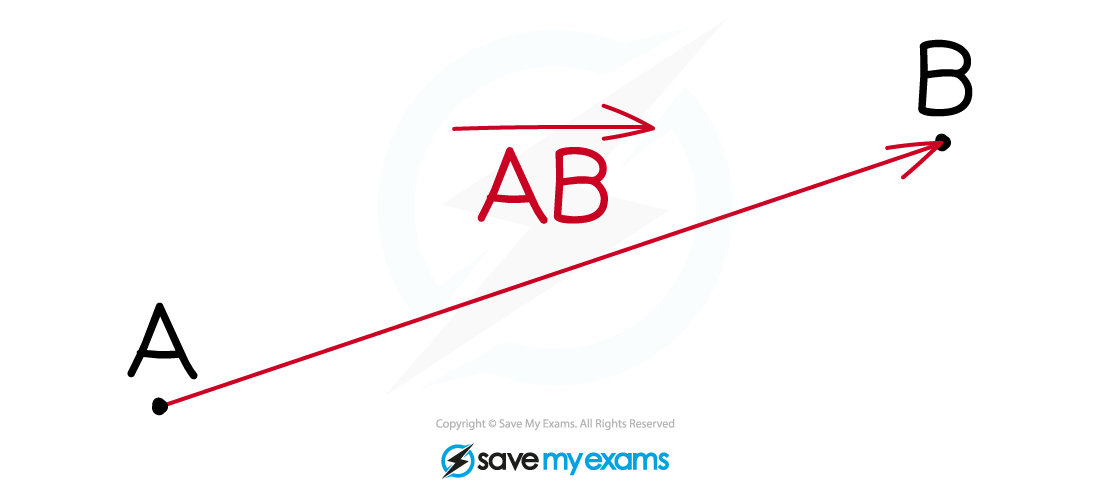• Note that the order of the letters is important! Vector BA in the above diagram would point in the opposite direction (ie with its ‘tail’ at point B, and the arrowhead at point A).

#### Vectors and transformation geometry

In transformation geometry, translations are indicated in the form of a column vector:• In the following diagram, Shape A has been translated six squares to the right and 3 squares up to create Shape B
• This transformation is indicated by the translation vector (6 3) :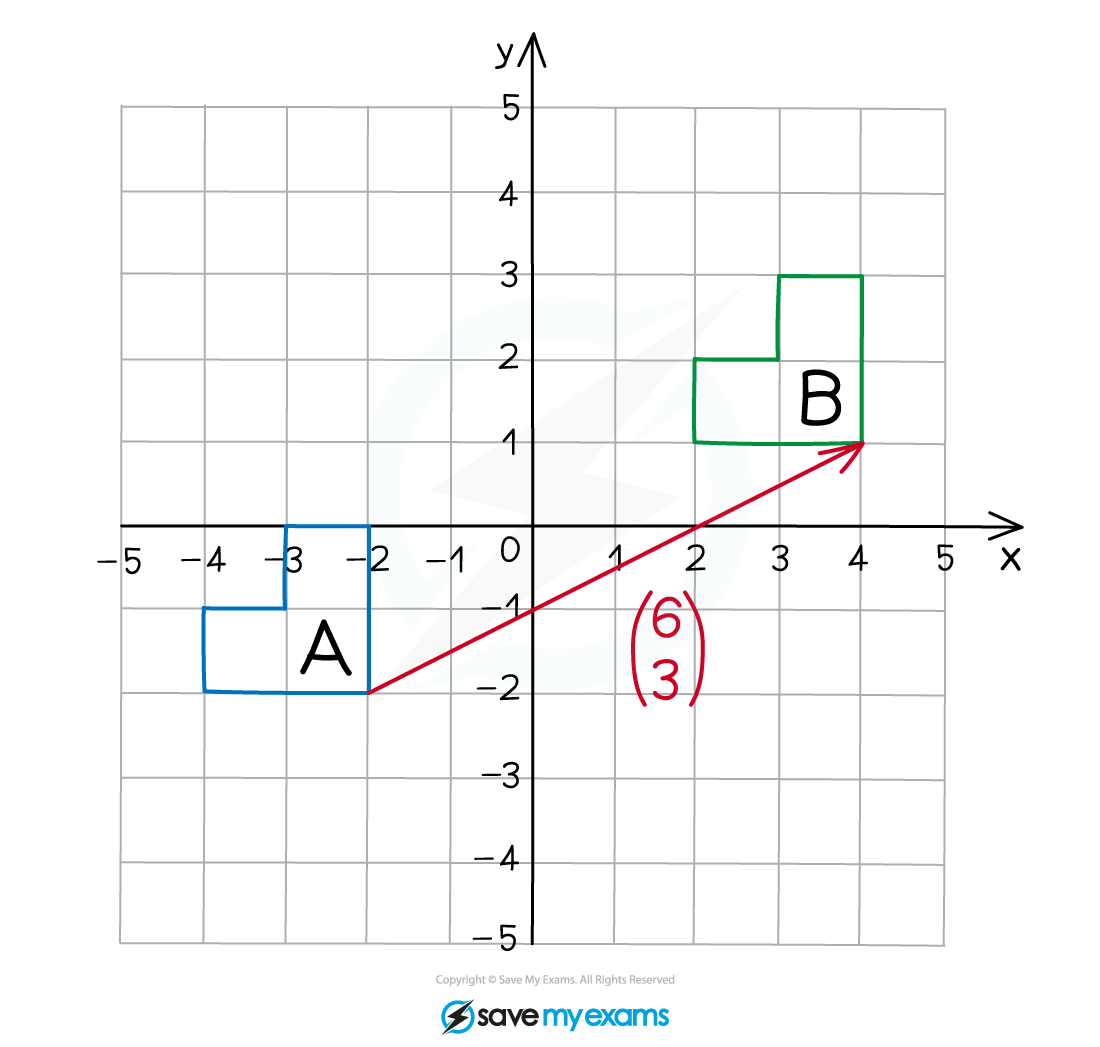• Note: ‘Vector’ is a word from Latin that means ‘carrier’
• In this case, the vector ‘carries’ shape A to shape B, so that meaning makes perfect sense!

#### Vectors on a grid

• You also need to be able to work with vectors on their own, outside of the transformation geometry context
• When vectors are drawn on a grid (with or without x and y axes), the vectors can be represented in the same (x y) column vector form as above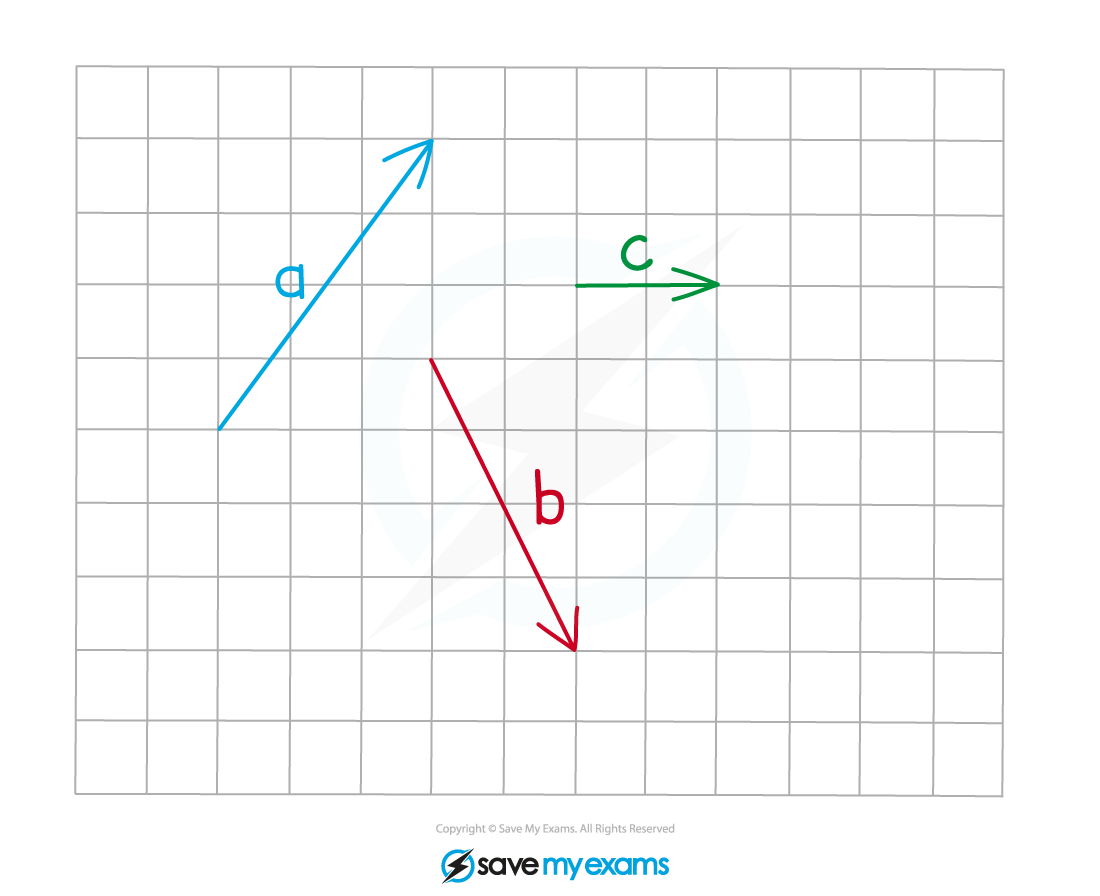a = (3 4)  b = (2 - 4)  c = (2 0)

#### Multiplying a vector by a scalar

• A scalar is a number with a magnitude but no direction – ie the regular numbers you are used to using
• When a vector is multiplied by a scalar, the magnitude of the vector changes, but its direction stays the same
• If the vector is represented as a column vector, then each of the numbers in the column vector gets multiplied by the scalar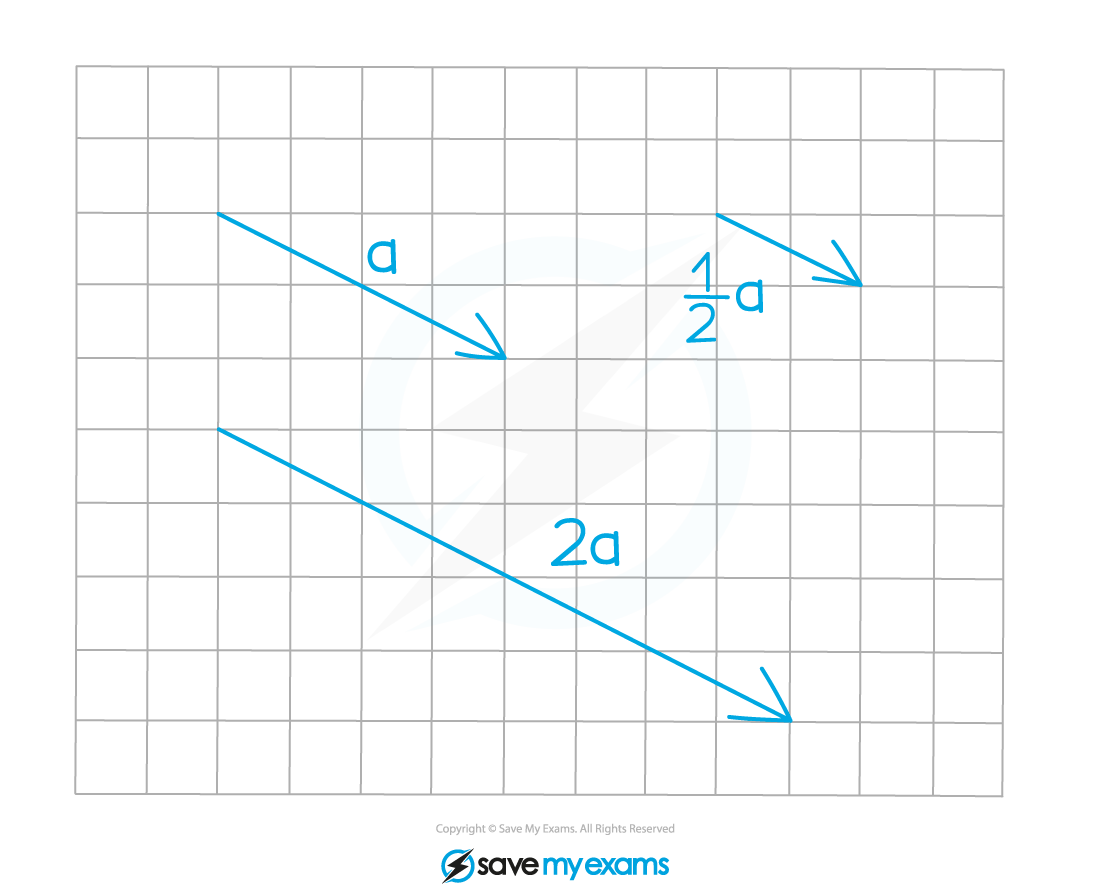a = (4 – 2)

2a = 2 × (4 - 2) = (2 × 4  2 × (-2)) = (8 - 4)

½a= ½ × (4 - 2) = (½ × 4  ½ × (-2)) = (2 - 1)

• Note that multiplying by a negative scalar also changes the direction of the vector: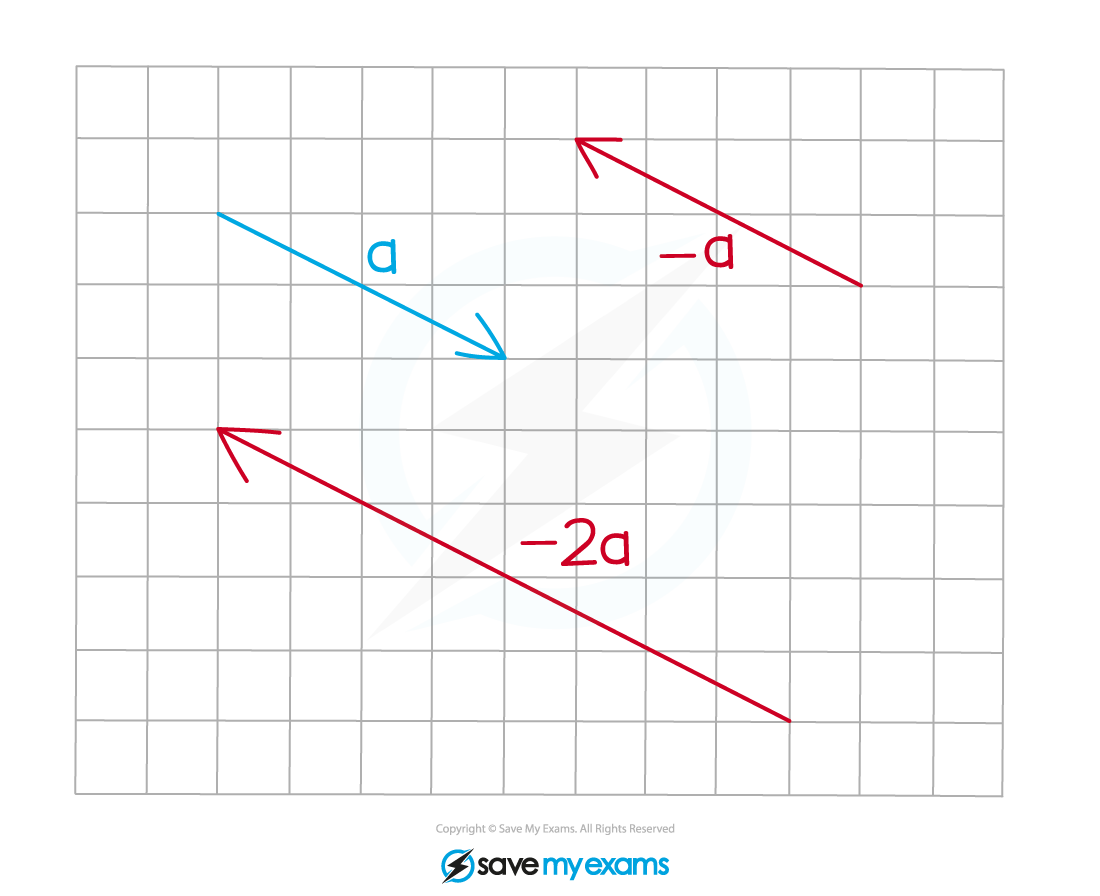a = (4 - 2)

-a = -1 × (4 - 2) = (-1 × 4  -1 × (-2)) = (-4 2)

-2a = -2 × (4 - 2) = (-2 × 4  - 2 × (-2)) = (-8 4)

• Note in particular that vector -a is the the same size as vector a, but points in the opposite direction!

• Adding two vectors is defined geometrically, like this: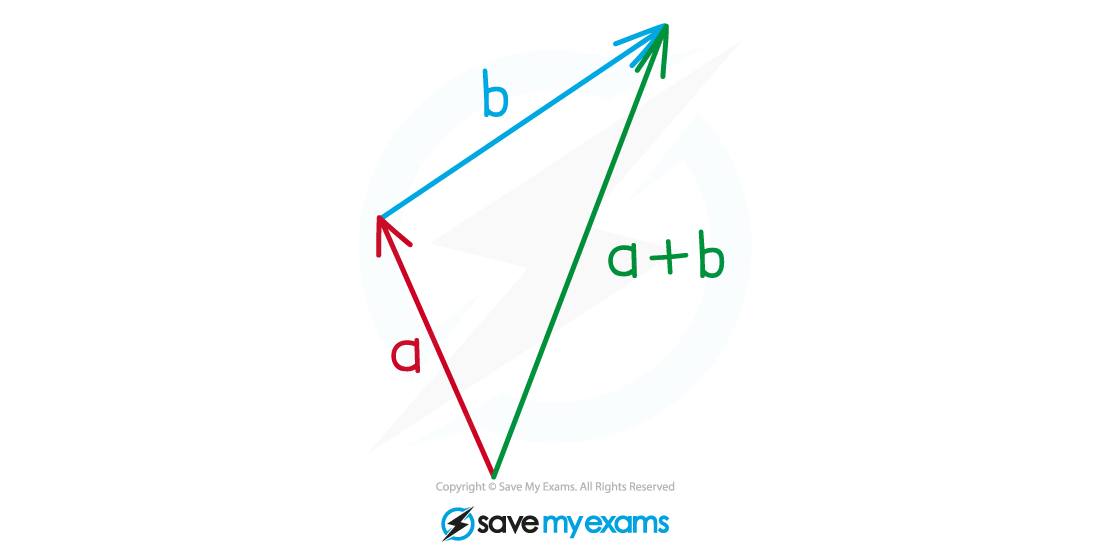• Subtracting one vector from another is defined as addition of the negative of the subtracted vector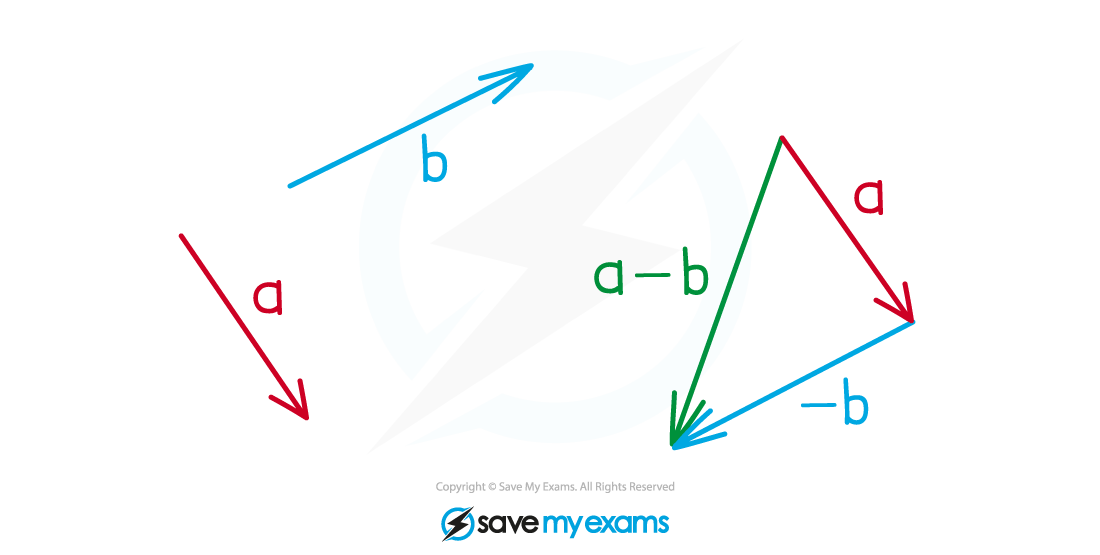a – b = a + (-b)

• When vectors are represented as column vectors, adding or subtracting is simply a matter of adding or subtracting the vectors’ x and y coordinates
• For example: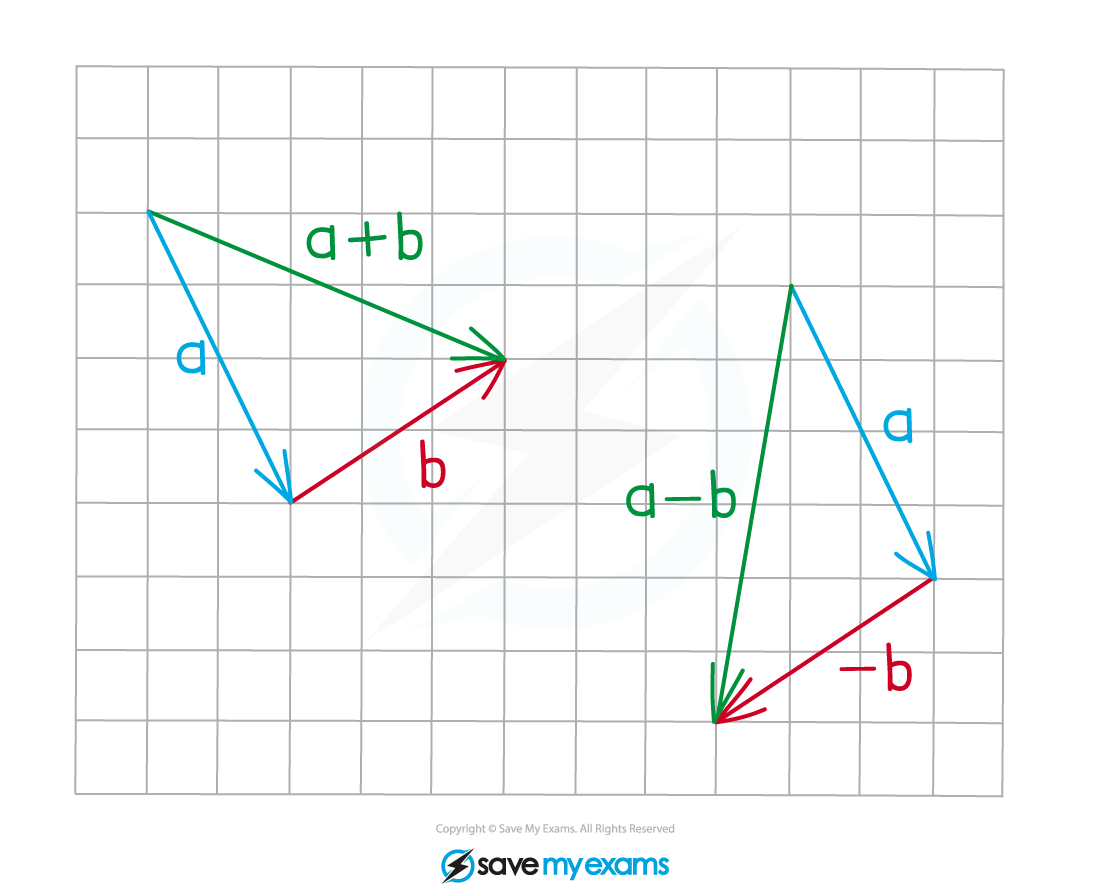a = (2 - 4)    b = (3 2)

a + b = (2 - 4) + (3 2) = (2 + 3  -4 +2) = (5 - 2)

a - b = (2 - 4) - (3 2) = (2 - 3  -4 -2) = (-1 - 6)

#### Worked Example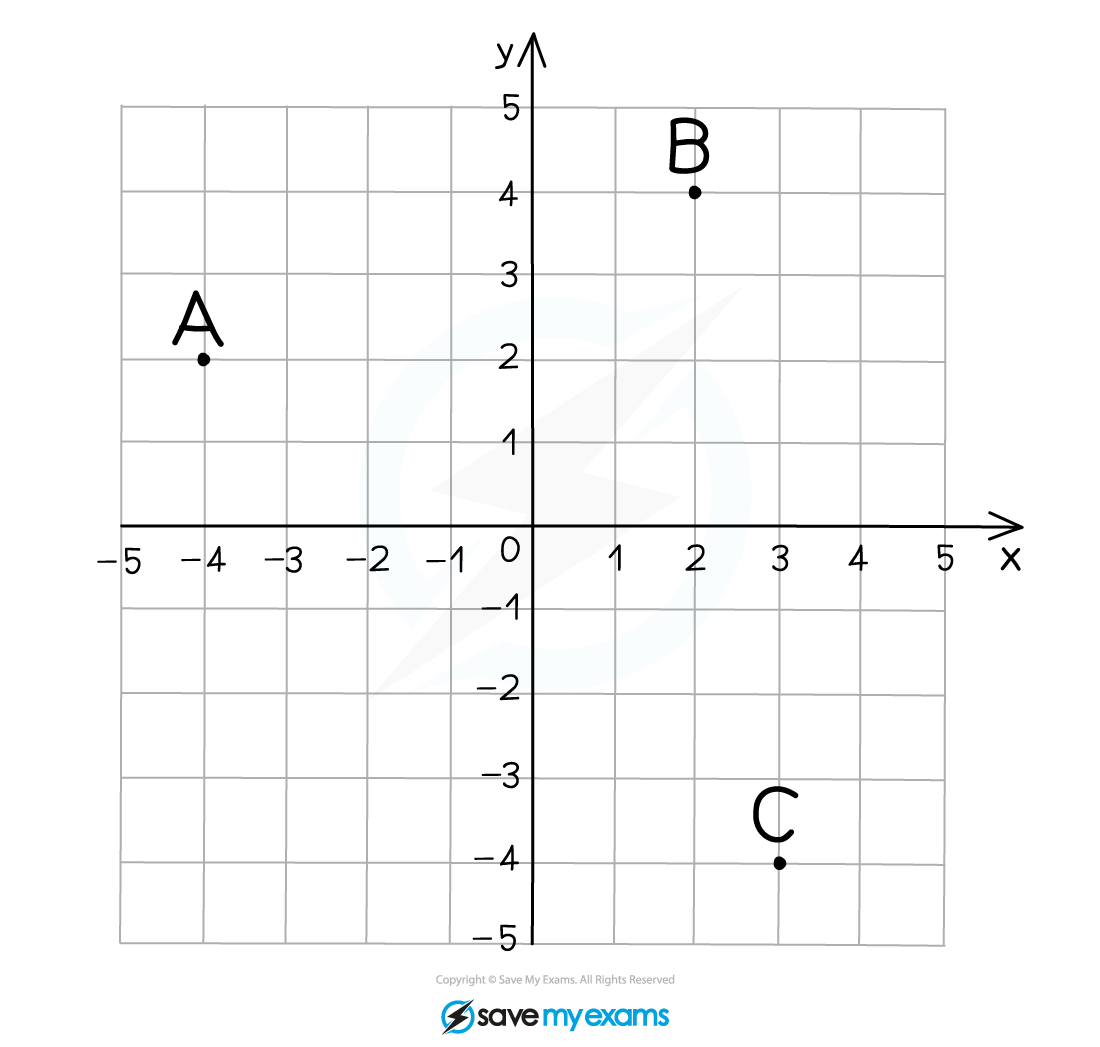#### Worked Example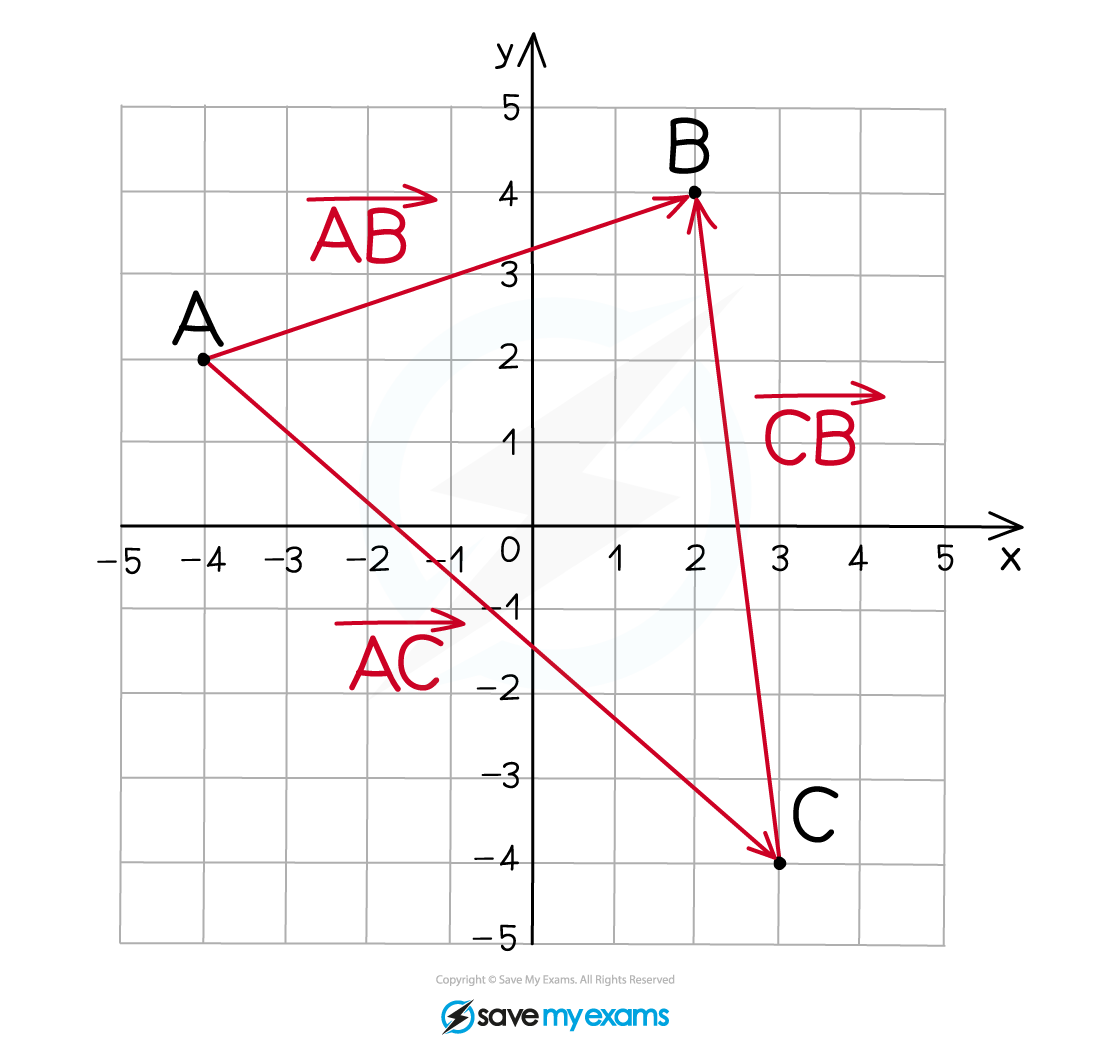#### Worked Example